# Clique decompositions of multipartite graphs and completion of Latin squares

A Latin square of order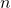is an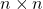grid of cells, each of which contains one ofdistinct symbols, such that no symbol appears twice in any row or column.  There is a natural correspondence between Latin squares of orderand partitions of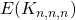into triangles.  We identify the three vertex classes of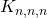with therows,columns andsymbols of the Latin square.  A triangle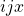corresponds to the symbol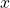appearing in the intersection of rowand column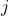of the Latin square.  Since each cell contains exactly one symbol, and each symbol appears exactly once in each row and each column, the triangles corresponding to a Latin square do indeed partition.

What about partitions of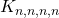into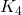‘s?  Identify the vertex classes ofwithrows,columns,red symbols andblue symbols.  Then a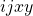corresponds to a red symboland a blue symbol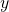in the intersection of rowand column.  If we look at just the red symbols or just the blue symbols then we see a Latin square.  But we also have the extra property that each pair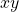of red and blue symbol appears in exactly one cell of the grid.  Two Latin squares with this property are called orthogonal.  So pairs of orthgonal Latin squares of ordercorrespond to decompositions ofinto‘s.  More generally, a sequence of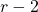mutually orthogonal Latin squares of ordercorresponds to a partition of the complete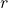-partite graph on vertex classes of sizeinto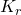‘s.

Suppose now that we have a partial Latin square of order, that is, a partially filled ingrid of cells obeying the rules for a Latin square.  Can it be completed to a Latin square?  In the early 1980s several researchers proved that the answer is yes provided at most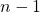cells have been filled in total.  This is best possible, as if we place‘s and a singleon the main diagonal there is no legal cell in which to place theth.  The same example shows that it is not enough to ask only that each row and each column contains only a small number of non-empty cells.  But what if each row, column and symbol has been used at most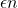times?  Can we then complete to a Latin square?  Daykin and Häggkvist conjectured that we can, provided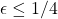.

What does this mean on the graph side?  Let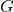be a subgraph ofobtained by deleting a set of edge-disjoint triangles such that no vertex is in more thantriangles.  Thenshould have a triangle-decomposition if.  In this paper we prove thathas a triangle-decomposition provided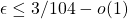.

In fact we prove something more general.  The‘s obtained in this way have the properties that (i) each vertex has the same number of neighbours in each other vertex class and (ii) each vertex sees at least a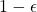proportion of the vertices in each other class (a partite minimum degree condition).  We prove that all such graphs have triangle-decompositions when.

The proof is based on that of the similar result for non-partite graphs in Edge decompositions of graphs with high minimum degree.  However, it is not simply a translation of that proof to the partite setting.  In the partite case we not only have to ensure that all of our gadgets can be embedded in partite graphs, we must also take care to ensure a stronger notion of divisibility is preserved throughout our decomposition process.  This makes the proof extremely technical.

We also prove the analogous result for-decompositions of complete-partite graphs, with the less impressive bound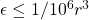.  The connection to mutually orthogonal Latin squares is more complicated for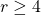, as the partially filled in cells only correspond to‘s in the case where each non-empty cell contains one of each symbol, but we still show that there is somesuch that if each row, column and coloured symbol is used at mosttimes then partial mutually orthogonal Latin squares can be complete.

As for the non-partite case, the bounds onare currently limited by available fractional or approximate decomposition results.  Improvements to these would lead automatically to improvements of the bounds in this paper.